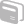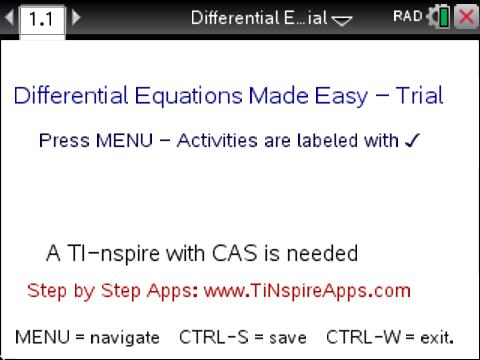# Activities

•• ##### Subject Area

• Math: Calculus: Differential Equations

• ##### Author9-12
College

• ##### Activity Time

10-15 Minutes

• ##### Device
•TI-Nspire™ CX CAS/CX II CAS
• ##### Software

TI-Nspire™ CX CAS

4.2

• ##### Report an Issue

Differential Equations Made Easy- Trial Edition#### Activity Overview

In Differential Equations Made Easy - Trial Edition, students will use TI-Nspire™ technology to explore differential equations problems utilizing step-by-step processes.

#### Vocabulary

• Differential equations
• Independent functions
• Determinant
• Laplace transform
• Wronskian

#### About the Lesson

In "Differential Equations Made Easy- Trial Version", there are five different activities for students to practice solving differential equations problems, using step-by-step processes.  To access the different activities within the TNS file, from the title screen, MENU >

• 1.Order DEQ> (4) Exact Differential Equation

Solve an Exact Differential Equation Step-by-Step: Learn how to solve an exact differential equation by plugging in an exact differential equation and optional initial conditions in the boxes, and then viewing the steps to find its solution below.

• 2.Order DEQ> (3) Homogenous Differential Equation

Compute Mean, Mode, Median: Learn how to solve a 2. order homogenous differential equation by plugging in the equation and optional initial conditions in the boxes, and then viewing the steps to find its solution below.

• Higher Order DEQ> (1) Linear Homogenous 3. Order DEQ

Solve a Linear 3. Order Differential Equation Step-by-Step: Learn how to solve a 3. Order differential equation by plugging in the equation and optional initial conditions in the boxes, and then viewing the steps to find its solution below.

• Transforms> (2)Laplace Transform

Perform Laplace Transform Step-by-Step: Learn how to perform Laplace Transform by plugging in the function in the t domain in the box, and viewing the steps to find its Laplace Transform below.

• Extras> (2) Find Wronskian

Find Wronskian Step-by-Step: Learn how to find Wronskian by plugging in the functions in the boxes and view the steps below to find the Wronskian, its determinant and conclusion if functions are independent or not.

• Note that all items with a check-mark next to it are functional in this trial version, so there are more activities available in the file to preview then those listed above. For more information on purchasing the full version, visit SMARTSOFT.